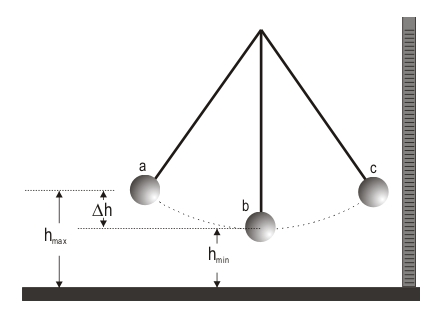# What is Measurement and Uncertainty?Measurement uncertainty can obscure science concepts like conservation of energy. Students need a solid foundation of measurement technique to be able to learn science.

Here is a common situation in today's inquiry-based science classroom: an instructor leads a lab activity that will demonstrate the concept of conservation of mechanical energy. Students measure the energy of a pendulum at various points during its swing to compare the total energy at various locations. No matter how careful they are, most students will measure different values for the energy of the pendulum at different locations. What does that mean? Is energy conserved or not? Some students will find the energy increases as the pendulum moves, for others it decreases.

A common scapegoat is the catch-all culprit "error." But what do we as instructors mean when we say error? Are we implying that students made a mistake? Are the variations in measurements really errors? To be able to make sense of this situation, students need a firm understanding of measurement uncertainty. They need to know how to determine the measurement uncertainty, and how to preserve measurement uncertainty during calculations. Finally, they need to be able to state results in terms of uncertainty. Given the trend towards teaching science by inquiry, students must be able to understand the role of measurement uncertainty when they use data to draw conclusions about science concepts.

Effective measurement technique includes these key concepts:

• Distinguishing between error and uncertainty
• Recognizing that all measurements have uncertainty
• Identifying types of error, sources of error and how to detect/minimize error
• Estimating, describing, and expressing uncertainty in measurements and calculations
• Using uncertainty to describe the results of their own lab work
• Comparing measured values and determine whether values are the same within stated uncertainty.

### Defining Error and Uncertainty

Some of the terms in this module are used by different authors in different ways. As a result, the use of some terms here might conflict with other published uses. The definitions used in this module are intended to match the usage in documents such as the NIST Reference on Constants, Units and Uncertainty.

For example, the term error, as used here, means the difference between a measured value and the true value for a measurement. Since the exact or "true" measured value of quantity can often not be determined, the error in a measurement can rarely be determined. Instead, it is more consistent with the NIST methods to quantify the uncertainty of a measurement.

Uncertainty as used here means the range of possible values within which the true value of the measurement lies. This definition changes the usage of some other commonly used terms. For example, the term accuracy is often used to mean the difference between a measured result and the actual or true value. Since the true value of a measurement is usually not known, the accuracy of a measurement is usually not known either. Because of these definitions, we modified how we report lab results. For example, when students report results of lab measurements, they do not calculate a percent error between their result and the actual value. Instead, they determine whether the accepted value falls within the range of uncertainty of their result.

The materials presented here are intended to teach measurement technique to students grades 9 through introductory college level. In addition, we have presented examples showing how to integrate these concepts into existing lab activities.